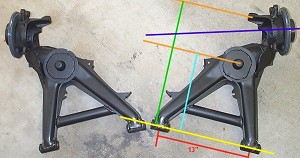Home Back to Tech Articles Back to Suspension Tech
 E30 Effective Wheel Rates
 Page 1 / Page 2
Some accurate measurementsReaders often e-mail me to say that they feel the derivation for wheel ratio on the previous page is wrong. They can't see why the motion ratio (ds/dw) should be squared. One's basic intuition says that this should not be required. The "fuzzy" way to think about it is like this:
You need to consider the motion ratio once to see how far the spring is compressed when you displace the wheel by some distance. Then you need to invoke the motion ratio a second time to transfer the force developed by the spring back out to the wheel. So the motion ratio is employed twice when converting spring rate to wheel rate, and hence the power of two on the motion ratio in the equation for wheel rate.

Once a reader is prompted to carefully read through the entire derivation on the previous page, a level of understanding is gained. Nonetheless, ever since I posted the "Effective Rate" page, I have been told by a couple of Norwegian E30 enthusiasts (Ove Kvam and Pĺl Berg) that my results are incorrect. However, Ove and Pĺl agreed with the derivation, they just did not agree with the measured values for the lever-arms. And hence they did not agree with the ultimate factors for converting spring ratio to wheel ratio.

So I finally forced myself to make the time to take some accurate measurements of the front and rear motion ratios on my E30 M3. That is, I removed the spring, put a jack under the hub, and took a series of data points of hub position vs. spring perch position. And indeed these measurements show that Ove and Pĺl were right, my numbers on the previous page were incorrect. The new data is presented in the following pair of links:

Data for REAR suspension wheel ratio.
Data for FRONT suspension wheel ratio.

Note that the spreadsheets average the results for each displacement measurement - thus assuming that the wheel rate is constant over the range of interest. There is, of course, no reason that this should be so. But here we are striving for a first order approximation, so it will suffice to average the results. The data yields a spring rate -to- wheel rate conversion factor of 0.45 for the rear suspension, and 0.88 for the front suspension. These values differ somewhat from those presented in the old table on the previous page.

If one prints out the picture of a rear E30 trailing arm as shown on this page and measures the appropriate lever arm lengths then a spring-to-wheel rate conversion ratio of 0.38 is obtained for the E30 rear suspension. However, the result is quite dependent on where one locates the (virtual) center of the contact patch. Thus I put more faith in the values obtained from the spreadsheets above (although the actual conversion factor is likely to be somewhere in-between).

So, without further adieu, I hereby present the updated table of spring-to-wheel rate conversion factors. It can be said with a high degree of confidence that these numbers are closer to the true values than those presented on the previous page:

 Motion Ratio Effective Wheel Rate Front 0.94 wheel rate = 0.88 x spring rate Rear 0.67 wheel rate = 0.45 x spring rate

 Page 1 / Page 2
 Home Back to Tech Articles Back to Suspension Tech